# M-accretive-operator

(diff) ← Older revision | Latest revision (diff) | Newer revision → (diff)

Letbe a real Banach space with dual spaceand normalized duality mapping(cf. also Duality; Adjoint space). An operator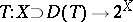is called accretive if for everyand everythere exists asuch that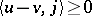(cf. also Accretive mapping). An accretive operatoris called-accretive if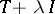is surjective for all(cf. also Surjection). Accretive and-accretive operators were introduced and studied intensively in the late 1960s and early 1970s. V. Barbu, F. Browder, H. Brézis, B.D. Calvert, M.G. Crandall, T. Kato, Y. Komura, A. Pazy, and S. Reich were among the first to recognize the importance and future impact of the class of-accretive operators.

There are two main problems associated with the class of-accretive operators: the time-dependent problem and the time-independent problem. The time-dependent problem is usually concerned with the solvability of first- and second-order evolution equations (cf. also Evolution equation), while the time-independent problem deals with equations of elliptic type (cf. also Elliptic partial differential equation). Some of the early highlights of the theory, related to the time-dependent problem, include: Kato's fundamental existence result (1967, [a8]), concerning the first-order evolution problem with a time-dependent-accretive operator in a Banach spacewith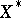uniformly convex (cf. also Banach space); the Crandall–Liggett theorem on generation of semi-groups (1971, [a4]) (in a general Banach space); and the Crandall–Pazy theorem on generation of an evolution operator (1972, [a5]), concerning the first-order evolution problem with a time-dependent-accretive operator in a general Banach space.

Spaceswithuniformly convex include all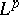spaces,. In [a8], T. Kato used the approximate evolution problem involving the Yosida approximants of the single-valued-accretive operator: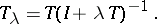In [a4], M.G. Crandall and T. Liggett made use, for the first time, of the classical method of lines in order to show the existence and the representation of the semi-group generated by an-accretive operatorin terms of a product formula. The method of lines was used again in [a5] to obtain the product formula of an evolution operator associated with the time-dependent problem. The class of time-dependent problems includes the class of problems involving functional-differential equations of the type(a1)

where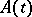is an-accretive operator. See [a9] for over one hundred references on these and other related problems, as well as for applications to the field of partial differential equations.

The elliptic-type problems often involve sums of time-independent operators, some of which may be accretive or-accretive. Several early results for sums of the type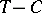, withaccretive andcompact, may be found in [a2]. In [a2], F. Browder developed degree theories for such operators (with strongly accretive mappings), whereis either continuous withuniformly convex, or uniformly continuous in a general Banach space. His construction of a degree mapping was based on the Leray–Schauder degree of the mappingon the set(cf. also Degree of a mapping), whereis an open set in. Actually, Browder's degrees can be constructed for more general mappings of the type, whereis a local homeomorphism. References to many later degree theories, as well a variety of other facts involving accretive and-accretive operators, can be found in [a7].

The theory of-accretive operators parallels the theory of maximal monotone operators in many ways. There are only a few basic properties of maximal monotone operators that do not yet have a counterpart in the class of the-accretive operators.

Naturally, the concept of an-accretive operator extends to complex Banach spaces, whereabove is replaced by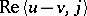.

How to Cite This Entry:
M-accretive-operator. Encyclopedia of Mathematics. URL: http://encyclopediaofmath.org/index.php?title=M-accretive-operator&oldid=17391
This article was adapted from an original article by A.G. Kartsatos (originator), which appeared in Encyclopedia of Mathematics - ISBN 1402006098. See original article